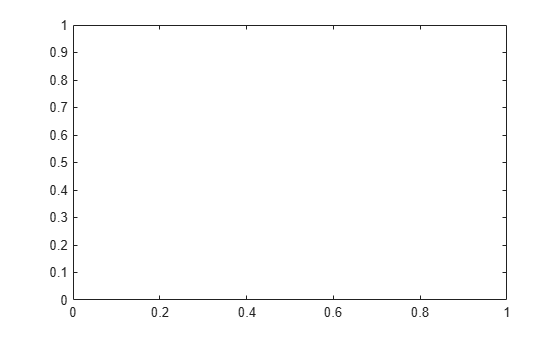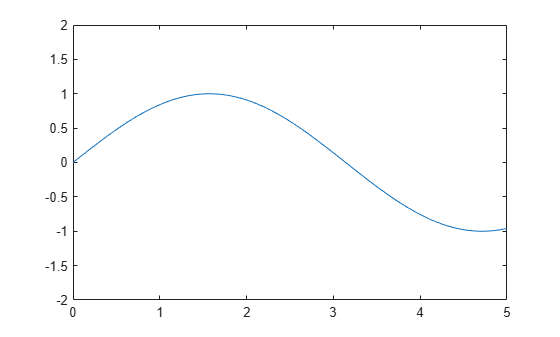Documentation

### This is machine translation

Mouseover text to see original. Click the button below to return to the English version of the page.

Note: This page has been translated by MathWorks. Click here to see
To view all translated materials including this page, select Country from the country navigator on the bottom of this page.

# cla

## Syntax

``cla``
``cla(ax)``
``cla reset``
``cla(ax,'reset')``

## Description

example

````cla` deletes all graphics objects that have visible handles from the current axes. The handle is visible if the `HandleVisibility` property of the object is set to `'on'`. The next plot added to the axes uses the first color and line style based on the `ColorOrder` and `LineStyleOrder` properties of the axes. If axes do not exist, then this command creates one.```

example

````cla(ax)` deletes graphics objects from the axes or polar axes specified by `ax` instead of the current axes.```

example

````cla reset` deletes graphics objects from the current axes regardless of their handle visibility. It also resets axes properties to their default values, except for the `Position` and `Units` properties.```

example

````cla(ax,'reset')` resets properties for the specified axes.```

## Examples

collapse all

Plot two sine waves. Then, clear the line plots from the axes.

```x = linspace(0,2*pi); y1 = sin(x); plot(x,y1) hold on y2 = sin(2*x); plot(x,y2)````cla``cla` clears the line plots and resets the `ColorIndex` and `LineStyleIndex` properties of the axes to 1. Subsequent plots start from the beginning of the color order and line style order. For example, plot another sine wave.

```y3 = sin(3*x); plot(x,y3) hold off```Create a figure with two subplots and assign the `Axes` objects to the variables `ax1` and `ax2`. Add plots to both axes.

```ax1 = subplot(2,1,1); surf(ax1,peaks) ax2 = subplot(2,1,2); contour(ax2,peaks)```Clear the surface plot from the upper subplot by specifying `ax1` as an input argument to `cla`.

`cla(ax1)`Now, reset all axes properties for the upper subplot, including the camera properties that control the view, by using the optional input argument `'reset'`.

`cla(ax1,'reset')`Create a line plot and set the axis limits.

```x = linspace(0,2*pi); y = sin(x); plot(x,y) axis([0 5 -2 2])```Clear the line plot from the axes and reset all the axes properties to their default values. `cla reset` resets all properties of the current axes, except for the `Position` and `Units` properties.

`cla reset`## Input Arguments

collapse all

Target axes, specified as an `Axes`object or a `PolarAxes` object. Use `ax` to clear specific axes, instead of the current axes.

## Algorithms

The `cla` command resets the `ColorOrderIndex` and `LineStyleOrderIndex` properties of the current axes to `1`.

Download ebook